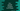# C++ program to convert 12 hours to 24 hours time format## C++ program to convert 12 hours to 24 hours time format:

In this post, we will learn how to convert 12 hours time to 24 hours time format in C++. This program will take the hours, minutes and seconds values as inputs from the user in 12 hours format and print it in 24 hours format.

For example, 12 AM is 00 hours in 24 hours format. Similarly, 12 PM is 12 hours in 24 hours format.

## Algorithm for the program:

We will follow the below algorithm:

• Initialze three integer variables to hold the hour, minute and second values.
• Also create one more string variable to hold the AM/PM value.
• Ask the user to enter the hour, minute, second and AM/PM values.

• If it is am and hour is 12, change it to 0 and print the time in 24 hours format.
• If it is pm and hour is less than 12, add 12 to the hour value and print the 24 hours format time.

That’s all. Now, let’s write down the program:-

## C++ program:

Below is the complete C++ program that converts a 12 hours time to 24 hours.

``````#include <iostream>
#include <iomanip>

using namespace std;

void print24hourTime(int hour, int min, int sec)
{
cout << setfill('0') << setw(2);
cout << hour << ":";

cout << setfill('0') << setw(2);
cout << min << ":";

cout << setfill('0') << setw(2);
cout << sec << endl;
}

void convert12to24(int hour, int min, int sec, bool am)
{
if (hour < 0 || hour > 12 || min > 60 || min < 0 || sec < 0 || sec > 60)
{
cout << "Invalid Time" << endl;
}
else if (am)
{
if (hour == 12)
{
print24hourTime(0, min, sec);
}
else if (hour < 12)
{
print24hourTime(hour, min, sec);
}
}
else
{
if (hour == 12)
{
print24hourTime(hour, min, sec);
}
else if (hour < 12)
{
print24hourTime(hour + 12, min, sec);
}
}
}

int main()
{
int hour, min, sec;
string median;

cout<<"Enter hour, min and seconds: "<<endl;
cin>>hour;
cin>>min;
cin>>sec;

cout<<"Enter AM/PM"<<endl;
cin>>median;

convert12to24(hour, min, sec, median == "AM");
}``````

### Explanation:

Here,

• hour, min and sec are three integer variables to hold the hour, minute and seconds. median is a string variable to hold the median value, i.e. AM or PM.
• convert12to24 method is used to convert the 12 hours format entered by the user to 24 hours. It takes four parameters, hour, minute, seconds and one boolean value that defines if it is AM or not.
• print24hourTime is used to print the time. It uses setw and setfill methods to make sure that it always prints the hour, minute and second values in two digits and for one digit, it will add one 0 to left.
• convert12to24 also validates the values. For any invalid hour, min or sec value, it will print one message.

### Sample output:

If you run this program, it will print output as like below:

``````Enter hour, min and seconds:
4
5
1
Enter AM/PM
AM
04:05:01

Enter hour, min and seconds:
12
11
30
Enter AM/PM
PM
12:11:30

Enter hour, min and seconds:
30
00
00
Enter AM/PM
AM
Invalid Time``````